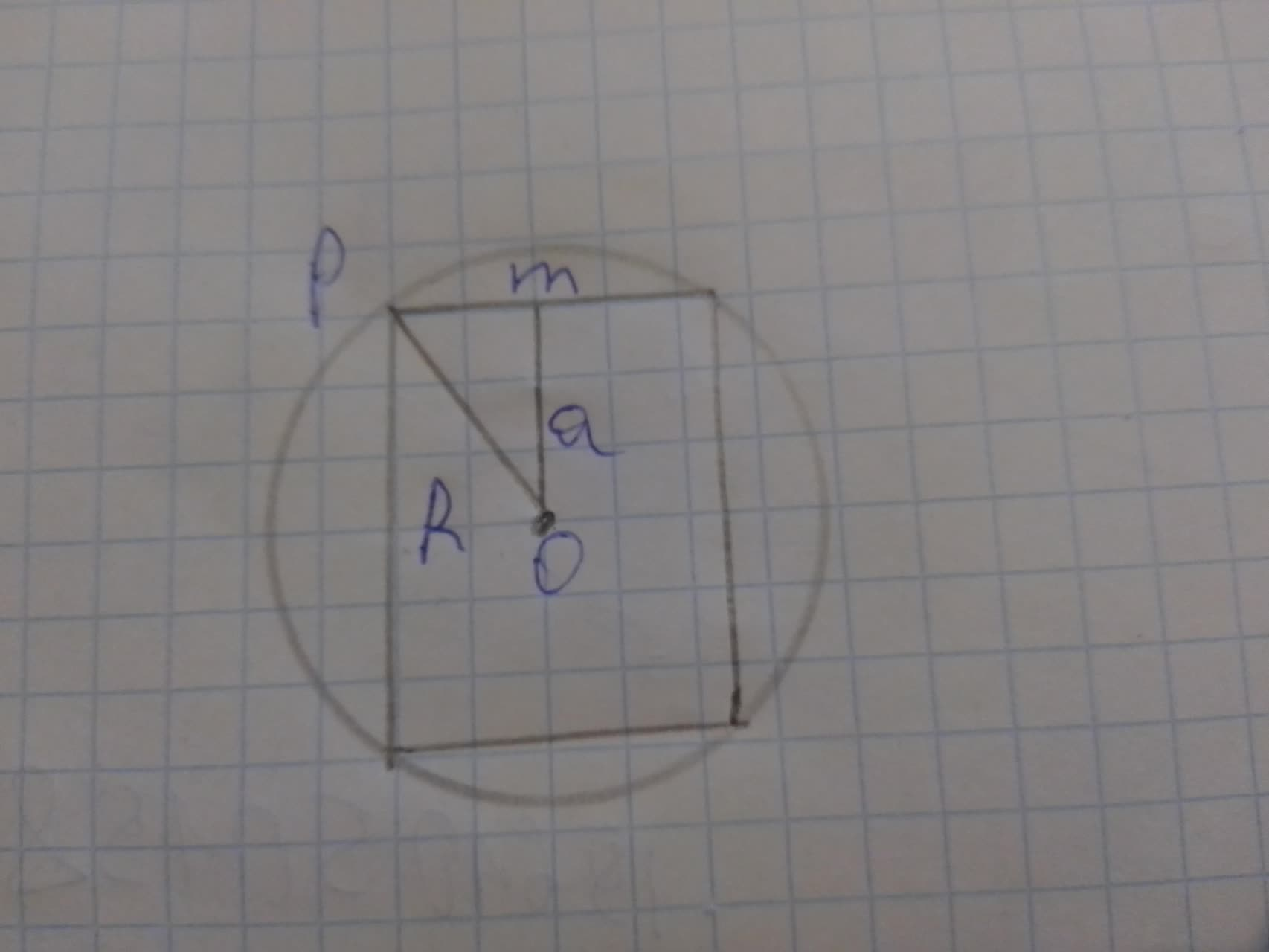Question# How do u find the radius of a circle with

Circles
ANSWEREDHow do u find the radius of a circle with a square inside that has the side lengths of 8cm.2021-08-09
Step 1
Topic- circles and square
Step 2$$\displaystyle{R}=?$$
here side of square
$$\displaystyle{a}={8}{c}{m}$$
$$\displaystyle\Rightarrow{o}{m}={1}{m}=\frac{{a}}{{2}}={4}{c}{m}$$
using pythagors theorem
$$\displaystyle{R}^{{{2}}}={\left({o}{m}\right)}^{{{2}}}+{\left(\pm\right)}^{{{2}}}$$
$$\displaystyle\Rightarrow{R}^{{{2}}}={\left({4}\right)}^{{{2}}}+{\left({4}\right)}^{{{2}}}$$
$$\displaystyle\Rightarrow{R}^{{{2}}}={32}$$
$$\displaystyle\Rightarrow{R}={4}\sqrt{{{2}}}{c}{m}$$